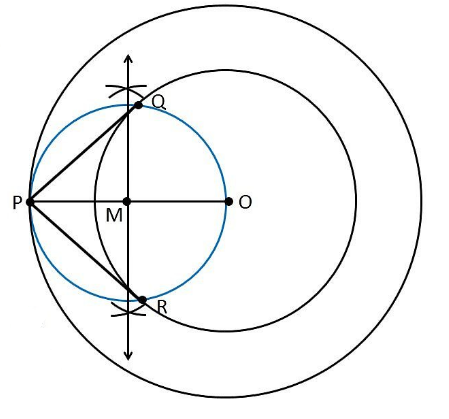Guru

# Construct a tangent to a circle of radius 4 cm from a point on the concentric circle of radius 6 cm and measure its length. Also verify the measurement by actual calculation. Q.2

• 1

What is the best way to solve the problem of question 2 of exercise 11.2 of constructions chapter of class 10th ncert math, how i solve this question Construct a tangent to a circle of radius 4 cm from a point on the concentric circle of radius 6 cm and measure its length. Also verify the measurement by actual calculation.

Share

1. Construction Procedure:

For the given circle, the tangent can be drawn as follows.

1. Draw a circle of 4 cm radius with centre “O”.

2. Again, take O as centre draw a circle of radius 6 cm.

3. Locate a point P on this circle

4. Join the points O and P through lines such that it becomes OP.

5. Draw the perpendicular bisector to the line OP

6. Let M be the mid-point of PO.

7. Draw a circle with M as its centre and MO as its radius

8. The circle drawn with the radius OM, intersect the given circle at the points Q and R.

9. Join PQ and PR.

10. PQ and PR are the required tangents.

From the construction, it is observed that PQ and PR are of length 4.47 cm each.

It can be calculated manually as follows

In ∆PQO,

Since PQ is a tangent,

∠PQO = 90°. PO = 6cm and QO = 4 cm

Applying Pythagoras theorem in ∆PQO, we obtain PQ2+QO2 = PQ2

PQ2+(4)2 = (6)2

PQ2 +16 =36

PQ2 = 36−16

PQ2 = 20

PQ = 2√5

PQ = 4.47 cm

Therefore, the tangent length PQ = 4.47Justification:

The construction of the given problem can be justified by proving that PQ and PR are the tangents to the circle of radius 4 cm with centre O.

To prove this, join OQ and OR represented in dotted lines.

From the construction,

∠PQO is an angle in the semi-circle.

We know that angle in a semi-circle is a right angle, so it becomes,

∴ ∠PQO = 90°

Such that

⇒ OQ ⊥ PQ

Since OQ is the radius of the circle with radius 4 cm, PQ must be a tangent of the circle. Similarly, we can prove that PR is a tangent of the circle.

Hence, justified.

• 1